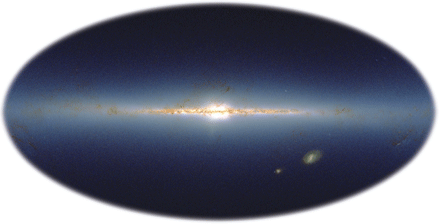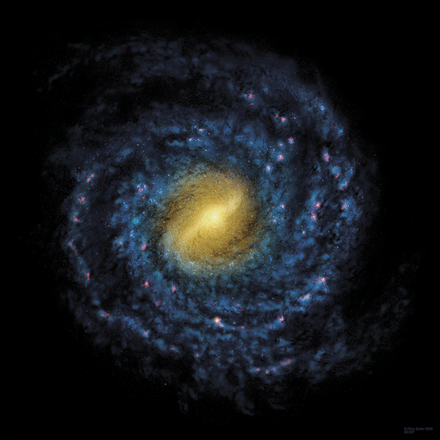1，在行为自身的层面；
2，进一步作为认知对象的层面。

## 作为场论的广义相对论

1.引力作为时空的曲率，度规张量:
$$g_{\mu\nu}(t,\vec{x})=g_{\nu\mu}$$
2.无质量自旋2粒子
$$h_{\mu\nu}=h_{\nu\mu}->$$
$$g_{\mu\nu}=\eta_{\mu\nu}+h_{\mu\nu}+\sigma(h^2)$$

## 黑洞里面可能的聚会

1.如何理解其事件地平线附近发生的事件?以及其事件地平线以内的宏观微观性质?
2.当引力物质积聚半径小于Schwarzschild半径之后,经典引力论下的无穷大密度奇点解与黑洞构成有限密度如何理解?

## 目前可见宇宙的砖块-碟子,引力以及自旋CREDIT: IPAC/CALTECH/2MASS/NASACREDIT: CHRIS BUTLER